# 1. Distance,_Displacement,_Speed,_and_Velocity.ppt

Mar. 31, 2023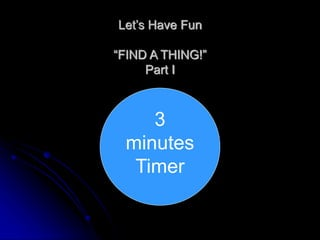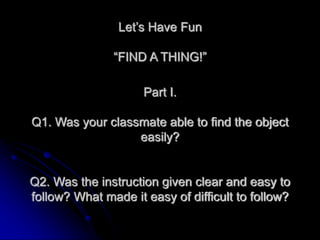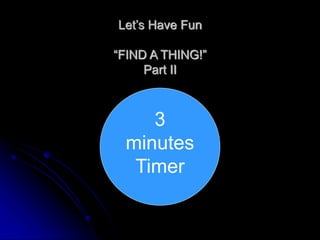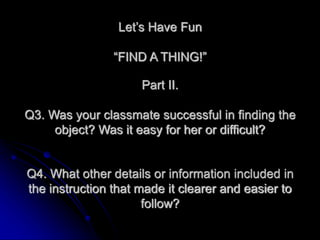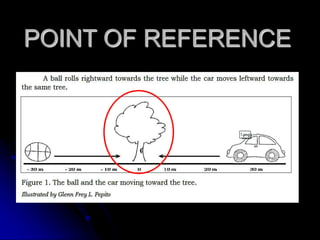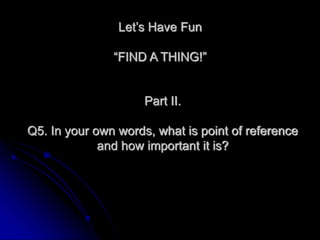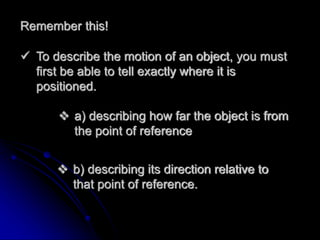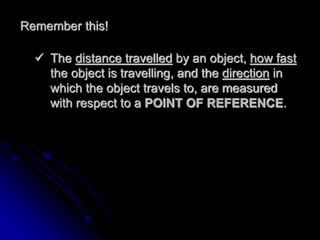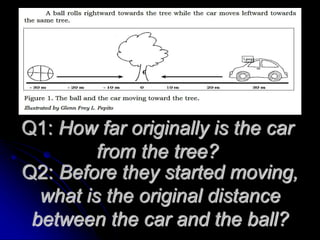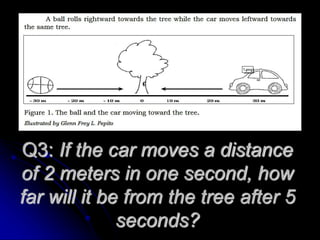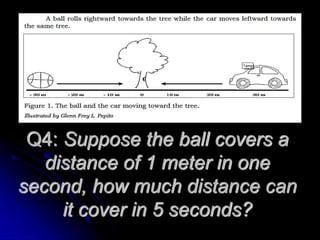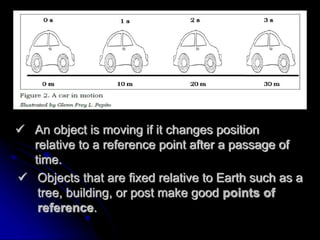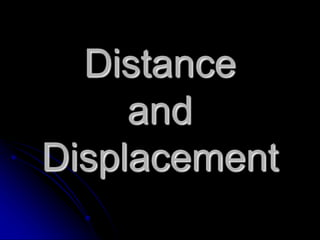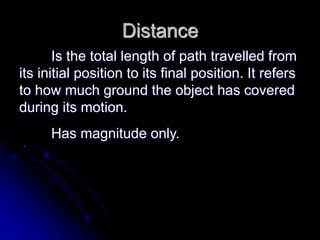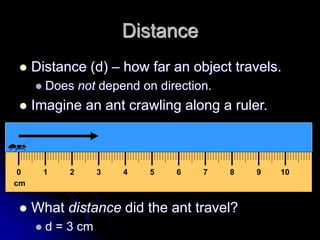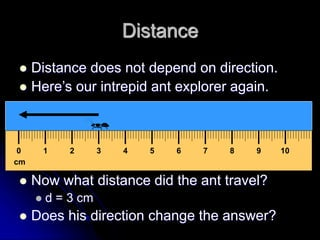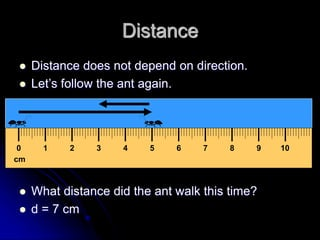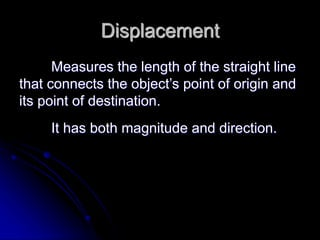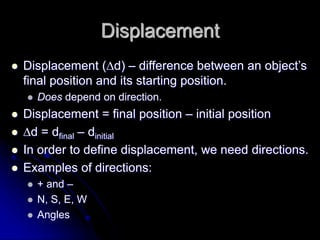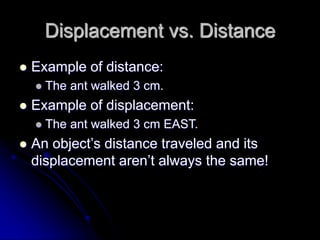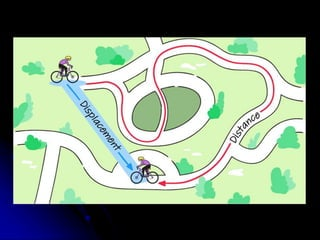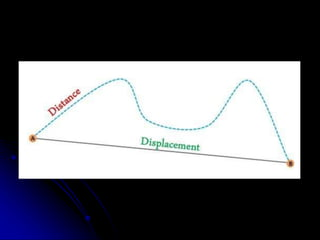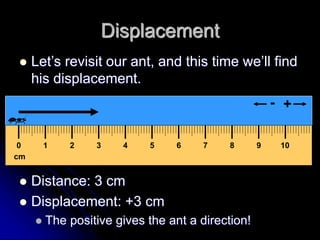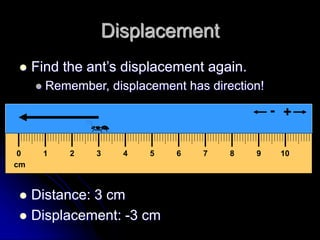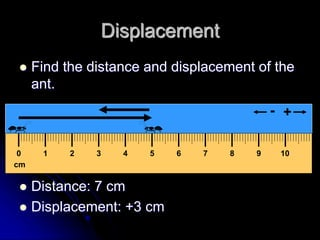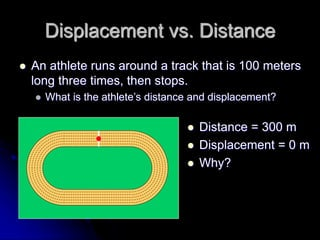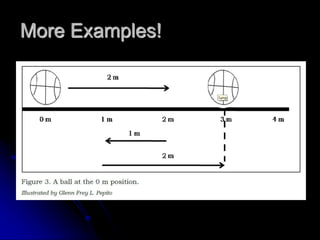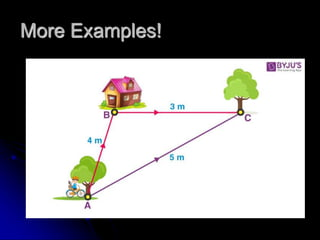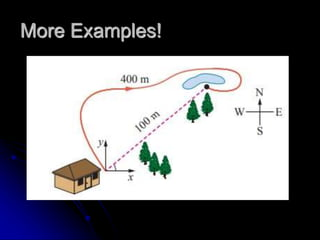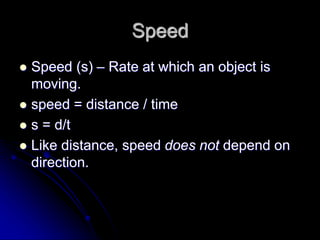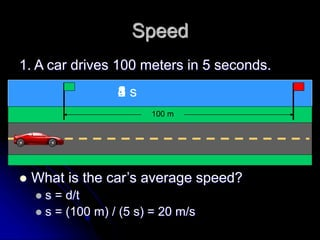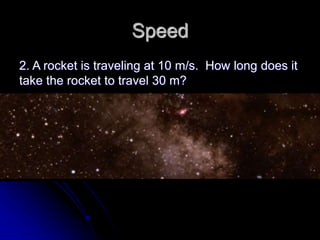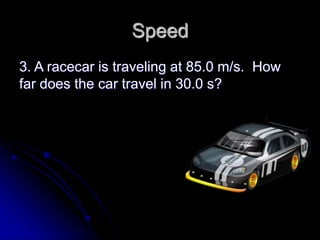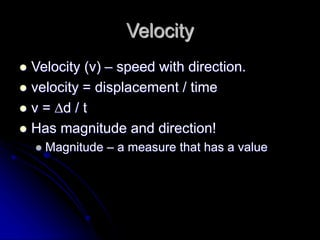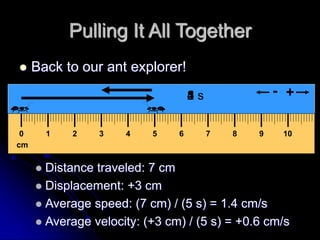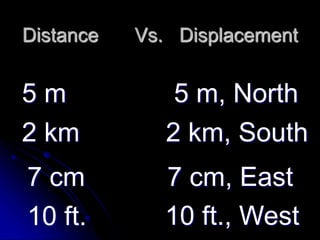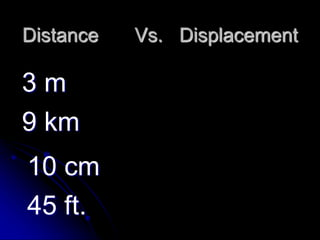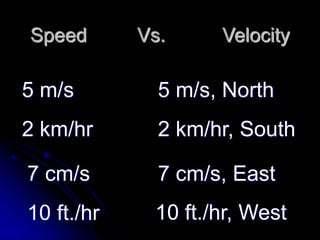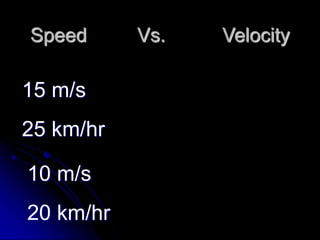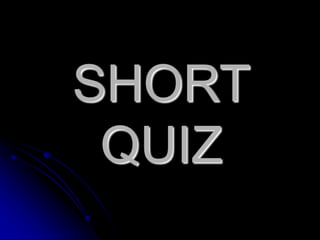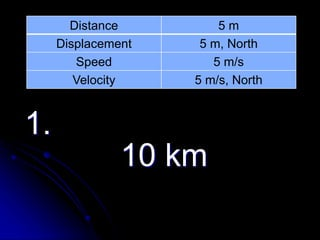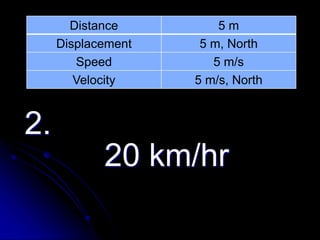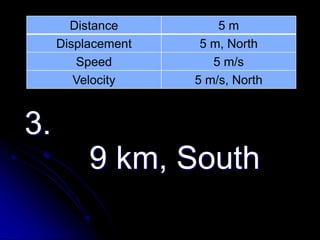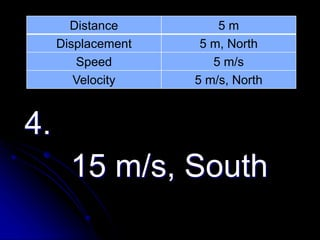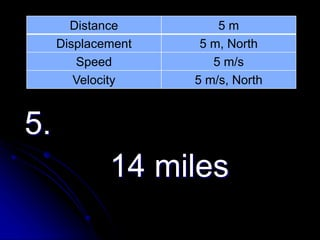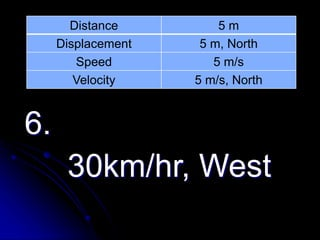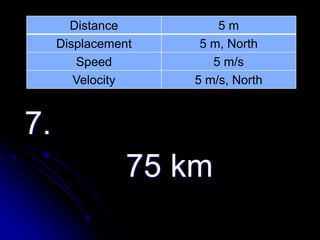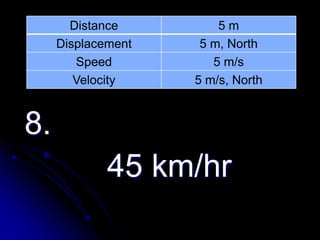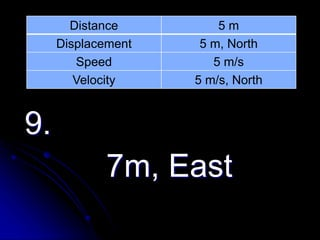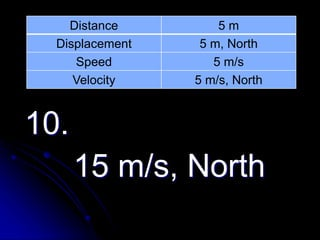1 of 51

### 1. Distance,_Displacement,_Speed,_and_Velocity.ppt

1. Let’s Have Fun “FIND A THING!” Part I 3 minutes Timer
2. Let’s Have Fun “FIND A THING!” Part I. Q1. Was your classmate able to find the object easily? Q2. Was the instruction given clear and easy to follow? What made it easy of difficult to follow?
3. Let’s Have Fun “FIND A THING!” Part II 3 minutes Timer
4. Let’s Have Fun “FIND A THING!” Part II. Q3. Was your classmate successful in finding the object? Was it easy for her or difficult? Q4. What other details or information included in the instruction that made it clearer and easier to follow?
5. POINT OF REFERENCE
6. Let’s Have Fun “FIND A THING!” Part II. Q5. In your own words, what is point of reference and how important it is?
7. Remember this!  To describe the motion of an object, you must first be able to tell exactly where it is positioned.  a) describing how far the object is from the point of reference  b) describing its direction relative to that point of reference.
8. Remember this!  The distance travelled by an object, how fast the object is travelling, and the direction in which the object travels to, are measured with respect to a POINT OF REFERENCE.
9. Q1: How far originally is the car from the tree? Q2: Before they started moving, what is the original distance between the car and the ball?
10. Q3: If the car moves a distance of 2 meters in one second, how far will it be from the tree after 5 seconds?
11. Q4: Suppose the ball covers a distance of 1 meter in one second, how much distance can it cover in 5 seconds?
12.  An object is moving if it changes position relative to a reference point after a passage of time.  Objects that are fixed relative to Earth such as a tree, building, or post make good points of reference.
13. Distance and Displacement
14. Distance Is the total length of path travelled from its initial position to its final position. It refers to how much ground the object has covered during its motion. Has magnitude only.
15. Distance  Distance (d) – how far an object travels.  Does not depend on direction.  Imagine an ant crawling along a ruler.  What distance did the ant travel?  d = 3 cm cm 0 1 2 3 4 5 6 7 8 9 10
16. Distance  Distance does not depend on direction.  Here’s our intrepid ant explorer again.  Now what distance did the ant travel?  d = 3 cm  Does his direction change the answer? cm 0 1 2 3 4 5 6 7 8 9 10
17. Distance  Distance does not depend on direction.  Let’s follow the ant again.  What distance did the ant walk this time?  d = 7 cm cm 0 1 2 3 4 5 6 7 8 9 10
18. Displacement Measures the length of the straight line that connects the object’s point of origin and its point of destination. It has both magnitude and direction.
19. Displacement  Displacement (d) – difference between an object’s final position and its starting position.  Does depend on direction.  Displacement = final position – initial position  d = dfinal – dinitial  In order to define displacement, we need directions.  Examples of directions:  + and –  N, S, E, W  Angles
20. Displacement vs. Distance  Example of distance:  The ant walked 3 cm.  Example of displacement:  The ant walked 3 cm EAST.  An object’s distance traveled and its displacement aren’t always the same!
21. cm 0 1 2 3 4 5 6 7 8 9 10 + - Displacement  Let’s revisit our ant, and this time we’ll find his displacement.  Distance: 3 cm  Displacement: +3 cm  The positive gives the ant a direction!
22. Displacement  Find the ant’s displacement again.  Remember, displacement has direction!  Distance: 3 cm  Displacement: -3 cm cm 0 1 2 3 4 5 6 7 8 9 10 + -
23. Displacement  Find the distance and displacement of the ant.  Distance: 7 cm  Displacement: +3 cm cm 0 1 2 3 4 5 6 7 8 9 10 + -
24. Displacement vs. Distance  An athlete runs around a track that is 100 meters long three times, then stops.  What is the athlete’s distance and displacement?  Distance = 300 m  Displacement = 0 m  Why?
25. More Examples!
26. More Examples!
27. More Examples!
28. That’s all for today!
29. Speed  Speed (s) – Rate at which an object is moving.  speed = distance / time  s = d/t  Like distance, speed does not depend on direction.
30. Speed 1. A car drives 100 meters in 5 seconds.  What is the car’s average speed?  s = d/t  s = (100 m) / (5 s) = 20 m/s 100 m 1 s 2 s 3 s 4 s 5 s
31. Speed 2. A rocket is traveling at 10 m/s. How long does it take the rocket to travel 30 m?
32. Speed 3. A racecar is traveling at 85.0 m/s. How far does the car travel in 30.0 s?
33. Velocity  Velocity (v) – speed with direction.  velocity = displacement / time  v = d / t  Has magnitude and direction!  Magnitude – a measure that has a value
34. Pulling It All Together  Back to our ant explorer!  Distance traveled: 7 cm  Displacement: +3 cm  Average speed: (7 cm) / (5 s) = 1.4 cm/s  Average velocity: (+3 cm) / (5 s) = +0.6 cm/s cm 0 1 2 3 4 5 6 7 8 9 10 + - 1 s 2 s 3 s 4 s 5 s
35. Distance Vs. Displacement 5 m 5 m, North 2 km 2 km, South 7 cm, East 7 cm 10 ft. 10 ft., West
36. Distance Vs. Displacement 3 m 9 km 10 cm 45 ft.
37. Speed Vs. Velocity 5 m/s 5 m/s, North 2 km/hr 2 km/hr, South 7 cm/s, East 7 cm/s 10 ft./hr 10 ft./hr, West
38. Speed Vs. Velocity 15 m/s 25 km/hr 10 m/s 20 km/hr
39. SHORT QUIZ
40. Distance 5 m Displacement 5 m, North Speed 5 m/s Velocity 5 m/s, North 1. 10 km
41. Distance 5 m Displacement 5 m, North Speed 5 m/s Velocity 5 m/s, North 2. 20 km/hr
42. Distance 5 m Displacement 5 m, North Speed 5 m/s Velocity 5 m/s, North 3. 9 km, South
43. Distance 5 m Displacement 5 m, North Speed 5 m/s Velocity 5 m/s, North 4. 15 m/s, South
44. Distance 5 m Displacement 5 m, North Speed 5 m/s Velocity 5 m/s, North 5. 14 miles
45. Distance 5 m Displacement 5 m, North Speed 5 m/s Velocity 5 m/s, North 6. 30km/hr, West
46. Distance 5 m Displacement 5 m, North Speed 5 m/s Velocity 5 m/s, North 7. 75 km
47. Distance 5 m Displacement 5 m, North Speed 5 m/s Velocity 5 m/s, North 8. 45 km/hr
48. Distance 5 m Displacement 5 m, North Speed 5 m/s Velocity 5 m/s, North 9. 7m, East
49. Distance 5 m Displacement 5 m, North Speed 5 m/s Velocity 5 m/s, North 10. 15 m/s, North